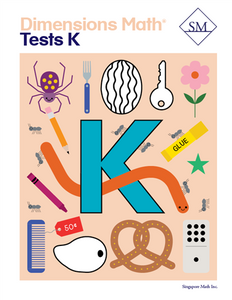# Dimensions Math Tests K

Vendor
Singapore Math Inc.
Regular price
\$13.70
Sale price
\$13.70
Quantity must be 1 or more

The Dimensions Math® PK-5 series is a new curriculum designed by Singapore Math Inc. to better serve U.S. teachers and students. Aspects of Singapore math curriculum have been updated for clarity and relevance, while preserving the solid foundation that makes it unique. The series follows the principles outlined in the Singapore Mathematics Framework and uses the Concrete > Pictorial > Abstract approach. The curriculum also incorporates Singapore mental math strategies and model drawing.

In the Dimensions Math program, concepts are introduced and explained in a new format with vibrant imagery. The implementation of this curriculum suits today’s needs, while the progression and scope that define Singapore math remain intact.

The Dimensions Math Tests books offer a series of assessment to help teachers and parents systematically evaluate student progress.

Samples of Dimensions Math Tests K

Kindergarten A

Test 1 - Chapter 1 Match, Sort, and Classify
Test 2 - Chapter 2 Numbers to 5 (Lessons 1–5)
Test 3 - Chapter 2 Numbers to 5 (Lessons 6–11)
Test 4 - Chapter 3 Numbers to 10 (Lessons 1–6)
Test 5 - Chapter 3 Numbers to 10 (Lessons 7-12)
Test 6 - Chapter 4 Shapes and Solids (Lessons 1–8)
Test 7 - Chapter 4 Shapes and Solids (Lessons 9–11)
Test 8 - Chapter 5 Compare Height, Length, Weight, and Capacity (Lessons 1–4)
Test 9 - Chapter 5 Compare Height, Length, Weight, and Capacity (Lessons 5–9)
Test 10-Chapter 6 Comparing Numbers Within 10

Kindergarten B

Test 11-Chapter 7 Numbers to 20 (Lessons 1–5)
Test 12-Chapter 7 Numbers to 20 (Lessons 6–9)
Test 13-Chapter 8 Number Bonds (Lessons 1–6)
Test 14-Chapter 8 Number Bonds (Lessons 7–11)
Test 15-Chapter 9 Addition (Lessons 1–6)
Test 16-Chapter 9 Addition (Lessons 7–11)
Test 17-Chapter 10 Subtraction (Lessons 1–5)
Test 18-Chapter 10 Subtraction (Lessons 6–9)
Test 19-Chapter 11 Addition and Subtraction
Test 20-Chapter 12 Numbers to 100 (Lesson 1-6)
Test 21-Chapter 12 Numbers to 100 (Lesson 7-10)
Test 22-Chapter 13 Time
Test 23-Chapter 14 Money